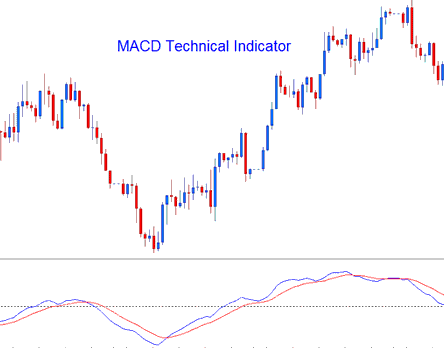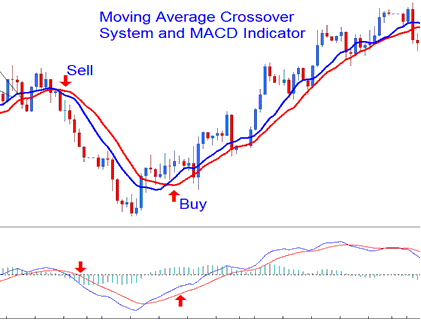# Moving Average Convergence/Divergence Stock Indices Technical Analysis and MACD Stock Indices Signals

Developed by Gerald Appel,

The Moving Average Convergence/Divergence is one of the simplest, reliable, and most commonly used indicators.

It is a momentum oscillator and also a trend-following indicator.

### Construction

The construction of this indicator calculates the difference between two moving averages and then plots that as the "Fast" line; the a second "Signal" line is then calculated from the resulting "Fast" line and then drawn on the same panel window as the "Fast" line.

• "Fast" line - Blue Line

• "Signal" line– Red Line

The "standard" MACD values for the "Fast" line is a 12-period exponential moving average and a 26-period exponential moving average and a 9-period exponential moving applied to the fast line, this plots the "Signal" line.

• Fast line= difference between 12 and 26 exponential moving averages

• Signal line= moving average of this difference of 9-periods## Stock Indices Technical Analysis and Generating Stock Indices Signals

The MACD is commonly used as a trend-following indicator and works most effectively when analyzing trending market movements. The three common methods of using MACD to generate signals are:

### Crossovers Signals:

Fast line/Signal line Crossover:

• A buy stock indices signal is generated when the Fast line crosses above the Signal line

• A sell stock indices signal is generated when the Fast line crosses below the Signal line.

However, in a strong trending market this stock indices signal gives a lot of whipsaws, the best crossover to use would thus be the Zero Line Crossover Signal that is less prone to whipsaws.

Zero Line Crossover Signals:

• When the Fast line crosses above zero center line a buy stock indices signal is generated.

• when the Fast line crosses below zero center line a sell stock indices signal is generated.### Divergence Stock Indices:

Looking for divergences between the MACD and stock index price can prove to be very effective in identifying potential reversal and/or stock indices trend continuation points in stock index price movement. There two types of divergences:

1. Classic Divergence Signals

2. Hidden Divergence Signals

### Overbought/Oversold Conditions:

MACD is also used to identify potential overbought/oversold conditions in stock index price action movements.

These levels are generated if the shorter MACD Lines separate dramatically from the median, this is an indication that stock index price action is over-extending & it will soon return to more realistic levels.

### MACD and Moving Average Crossover System

This indicator can be combined with others to form a stock index trading system. A good combination with the Moving Average crossover system. A trading signal is generated when both give a signal in the same direction.Technical Analysis